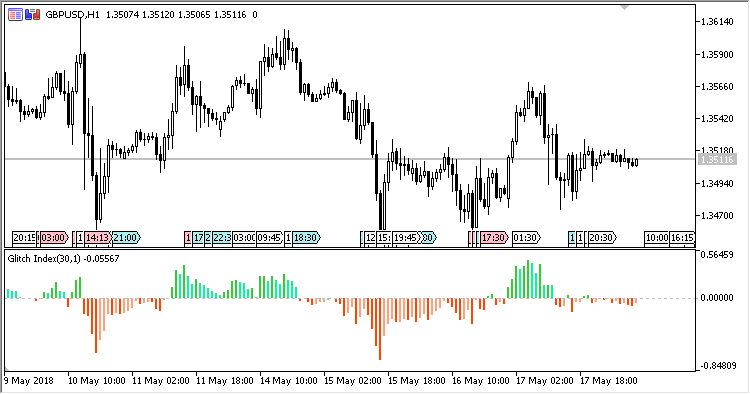# Glitch_Index – indicator for MetaTrader 5

• A+
category：MT5 INDICATORS

Glitch_Index is an indicator that shows the index of the price deviation from its average value. It was published in the February issue of Active Trader Magazine in 2004.

It has four input parameters:

• MA Period - MA period;
• MA method - MA calculation method;
• ROC Period - period of calculating the price rate of change (ROC);
• Applied price - price used for calculations.

Calculations:

`Glitch Index[i] = 100 * diff[i] / Price[i]`

where:

```diff[i] = Applied price[i] - smamult[i]
smamult[i] = MA(i)*((MA(i) - MA(i-ROC Period)) * 0.1+1)
```

MA - moving average with the calculation period of MA Period and the calculation method of MA method.